# Mass Parabola

In nuclear physics, a "mass parabola" refers to a graph of the binding energy per nucleon as a function of the atomic mass number.

The binding energy is the energy required to separate all the nucleons (protons and neutrons) in a nucleus. The binding energy per nucleon is a measure of the stability of the nucleus and is related to the amount of energy that would be released if the nucleus were to undergo a nuclear reaction.

When the binding energy per nucleon is plotted as a function of the atomic mass number, the resulting curve has a parabolic shape, known as the "mass parabola." The curve reaches a maximum at a certain atomic mass number, which corresponds to the most stable nuclei.

The mass parabola is a useful tool for understanding nuclear structure and for predicting the stability of unknown nuclei. By analyzing the shape of the curve, physicists can determine which nuclei are likely to undergo nuclear reactions, and which are likely to be stable.

The semi imperical mass parabola helps to account for some of the important features of stability of nucleus in particular 𝛽 activity and stability properties of isobars.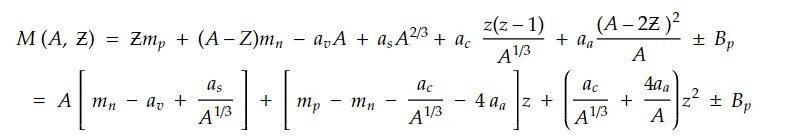For odd A nuclei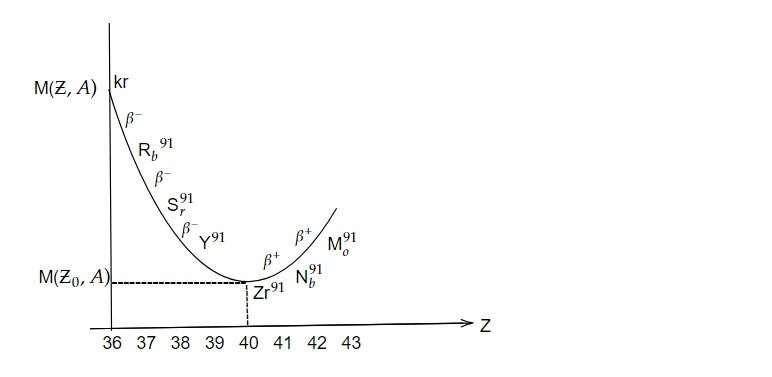For odd A, 𝛿 = 0, so there is only one parabola implying that there is only one stable nucleus. The mass M vs is plotted for a serial of nuclides with constant odd A = 91 and varying Ƶ. We see that most stable nucleus is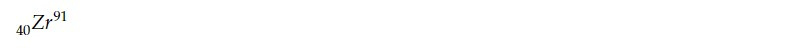Isobars to left of the stable nucleus have fewer protons (i.e larger mass) than stable one and decay by 𝛽- emission i.e.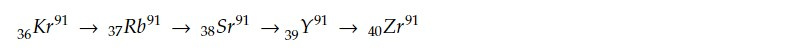Isobars to sight of stable isobars have excess proton than stable one and decay by 𝛽+ emission.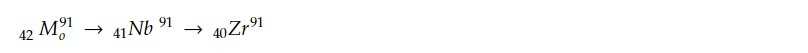For stable isobars z = Ƶo , 𝛽 + 2𝛾Ƶo = 0

=> Ƶo = - 𝛽 / 2𝛾 ......2

Putting 2 in 1,

M = 𝛼A + Ƶo (- 2𝛾Ƶo ) + 𝛾Ƶo²

= 𝛼A - 2𝛾Ƶo² + 𝛾Ƶo² = 𝛼A - 𝛾Ƶo²

Energy released during 𝛽- emission,Energy released during 𝛽+ emission,For even A nuclei

For even A, 𝛿 0, so there is two parabola displaced in Binding energy. 44Ru can't 𝛽 decay to 45Rh as mass of 45Rh>44Ru. Pd is stable having least mass in the family.

If Ru decays into Rh in a time, Δt = ℏ / ΔE, then violation of conservation of energy by an amount ΔE is consistent with Heisenberg's uncertainty principle and provides Rh decays to Pd within this time. i.e a double 𝛽 decay leading Ru to Pd is possible. Normally, time required for normal 𝛽 decay lis many second so probability for decay taking place in 10^-23 sec is extremely small and yet not observed experimentally.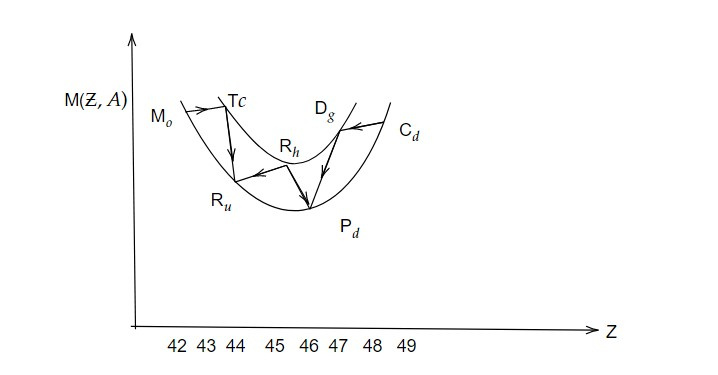Significance of Mass parabola

For odd N and odd Z, nuclei lies on upper curve and are therefore unstable with respect to those on lower curve, with the result that no stable odd odd nuclei should exist. The only exception to this rule are extremely light nuclei. For example isobaric family with A=14. In this family only 7N14 is stable but it lies on upper curve (odd-odd parabola). This emphasizes the fact that the light nuclei shouldn't be treated on the basis of Liquid drop model.

Therefore, the mass parabola in nuclear physics has several significant implications and applications. Here are some of its key significance:

1. Stability and Binding Energy: The shape of the mass parabola provides insights into the stability and binding energy of atomic nuclei. Nuclei with the highest binding energy per nucleon, corresponding to the peak of the parabola, are the most stable. This information is crucial for understanding nuclear stability and predicting the existence of stable and long-lived isotopes.

2. Nuclear Reactions: The mass parabola helps in predicting and understanding nuclear reactions. Nuclei that lie above or below the mass parabola can undergo nuclear reactions, such as radioactive decay or nuclear fission/fusion, to move towards the region of higher binding energy. The parabola serves as a guide for identifying potential nuclear reactions and studying their energetics.

3. Nuclear Structure: The mass parabola provides insights into the overall structure of atomic nuclei. It reveals patterns and trends in the binding energy as a function of atomic mass number, allowing for the identification of nuclear shells and subshells. These shell structures are analogous to electron shells in atoms and play a crucial role in determining the nuclear properties and behaviour.

4. Isotope Prediction: By extrapolating the mass parabola, physicists can make predictions about the existence and stability of unknown isotopes. The parabolic nature of the curve suggests the possibility of stable or long-lived isotopes in regions of the chart of nuclides that have not been experimentally observed yet. These predictions guide experimental efforts to synthesize and study new isotopes.

5. Nuclear Astrophysics: The mass parabola is relevant in nuclear astrophysics for understanding stellar nucleosynthesis, the process by which elements are formed in stars. It helps in identifying the most abundant isotopes in different stellar environments and provides insights into the nuclear reactions and processes occurring in stars.

In summary, the mass parabola in nuclear physics is a fundamental tool that helps in understanding nuclear structure, stability, reactions, and predicting the existence of isotopes. Its significance lies in its ability to provide a systematic and visual representation of the binding energy and properties of atomic nuclei.

Previous note: Nuclear Physics

This note is a part of the Physics Repository.